# OliNo

Renewable Energy

## Osram Lumilux L36W/840 double in luminaire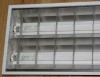This article gives interesting light parameters of lighting scheme that occurs quite often: two 1200 mm FL tubes in a build in luminaire. It serves as a nice comparison article for the newer type of LED-TL light tubes. An Eulumdat file is added in this article.

Note that a brandname has been chosen, Osram, so that theresults are comparable also to other TFL tubes of known brand names.

See this overview for a comparison with other light bulbs.

### Summary measurement data

parameter meas. result remark
Color temperature 3927 K Neutral white
Luminous intensity Iv 1791 Cd
Beam angle 88 deg
Power P 88 W
Power Factor 0.96 For every 1 kWh net power consumed, there has been 0.3 kVAhr for reactive power.
Luminous flux 3362 lm Osram reports that a single tube generates 3350 lm. In this article there are two tubes in a build-in luminaire. So the tubes should give 6700 lm, and only 50 % is retrieved as useful output and the other part is lost due to reflections and absorbation. This is quite common for build-in luminaires.
Luminous efficacy 38 lm/W
CRI_Ra 81 Color Rendering Index.
Coordinates chromaticity diagram x=0.4672 and y=0.4125
Fitting FL-tube
L x W external dimensions 1230 x 295 mm External dimensions of the luminaire.
L x W luminous area 1170 x 250 mm Dimensions of the luminous area (used in Eulumdat file). This is equal to the size of the opening of the inner side of the luminaire.
General remarks The ambient temperature during the whole set of measurements was 25.5-28 deg C.

Warm up effect: it takes 3 minutes for the tubes to give their full ight. The variation in ambient temperature results in little variation of the illuminance for thee FL tubes.

Voltage dependency: there is a linear dependency of  illuminance and power consumption on the voltage.

The Eulumdat file can be found via this link.

### Eulumdat light diagram

An interesting graph is the light diagram, indicating the intensity in the C0-C180 and the C90-C270 plane. This light diagram below comes from the program Qlumedit, that extracts these diagrams from an Eulumdat file.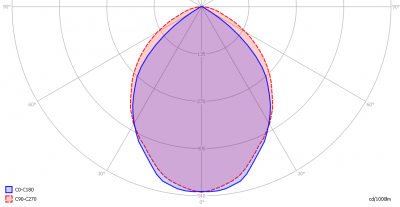The light diagram giving the radiation pattern.

It indicates the luminous intensity around the light bulb. The C0-C180 plane is perpendicular to the length direction of the tube. The C90-C270 is along the length direction.

The beam in the C0-C180 plane is slightly smaller than the beam in the C90-C270 plane.

The unit is Cd/1000lm, meaning the intensity in Cd assuming there would be 1000 lumen in the measured light bulb. This enables comparing different types of light bulbs.

### Illuminance Ev at 1 m distance, or luminous intensity Iv

Herewith the plot of the averaged luminous intensity Iv as a function of the inclination angle with the light bulb.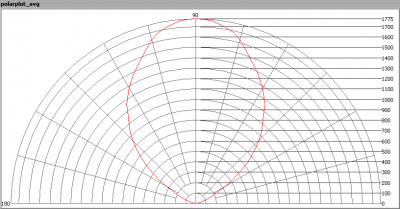The radiation pattern of the light bulb.

This radiation pattern is not the same as the one given earlier. This is because of the (small) differences of beam angles in the different planes. In this graph the luminous intensity is given in Cd.

These averaged values are used (later) to compute the lumen output.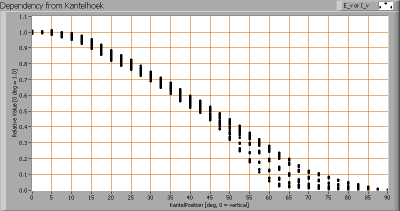Intensity data of every measured turn angle at each inclination angle.

This plot shows per inclination angle the intensity measurement results for each turn angle at that inclination angle.  There is some more spread in intensity results at higher inclination angles, when the lluminance is close to zero.

As the average values of illumination per inclination angle will be used for further computations, there is no effect on the further results.

When using the average values per inclination angle, the beam angle can be computed, being 88 degrees.

### Luminous flux

With the averaged illuminance data at 1 m distance, taken from the graph showing the averaged radiation pattern, it is possible to compute the luminous flux.

The result of this computation for this light spot is a luminous flux of 3362 lm.

### Luminous efficacy

The luminous flux being 3362 lm, and the power of the lightbulb being 88 W, yields a luminous efficacy of 38 lm/W.

A power factor of  0.96 means that for every 1 kWh net power consumed, a reactive component of 0.3 kVAr was needed.

 Light bulb voltage 230 V Light bulb current 400 mA Power P 88 W Apparent power S 92 VA Power factor 0.96

Of this light bulb the voltage across ad the resulting current through it are measured and graphed.Voltage across and current through the lightbulb
The current has its phase a bit lagging to the voltage (since a ballast is used, meaning the current will lag the voltage). Its form looks like a sine however. As there is little lag and as the current has a form like a since, the power factor is close to 1.
Also the power spectrum of the current is determined.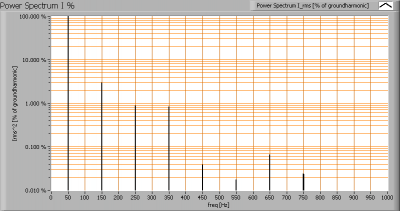The powerspectrum of the current through the light bulb.

There are some higher harmonics apparent in the spectrum.

### Color temperature and Spectral power distribution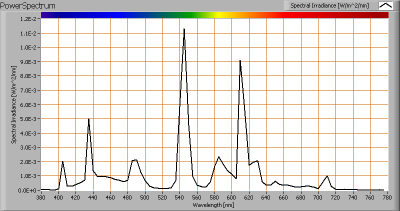The spectral power distribution of this light bulb.

The measured color temperature is about 3950 K which is neutral white.

This color temperature is measured straight underneath the light bulb. Below a graph showing the color temperature for different inclination angles.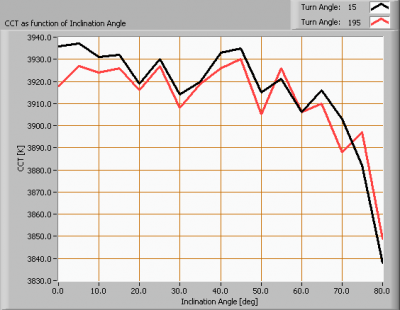Color temperature as a function of inclination angle.

The colortemperature is given for inclination angles up to 80 degrees since at larger inclination angles there is too little illumination.

The value remains constant within 1 % for angles up to 70 deg. After that the temperature drops sharply.

### Chromaticity diagram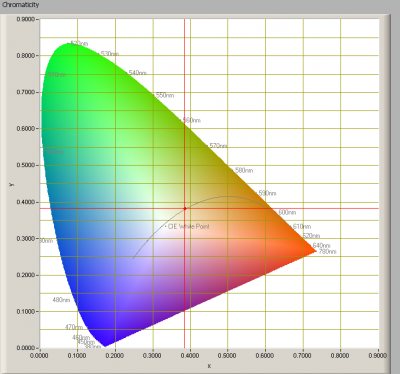The chromaticity space and the position of the lamp’s color coordinates in it.

The light coming from this lamp is on top of the Planckian Locus (the black path in the graph).

Its coordinates are x=0.3846 and y=0.3836.

### Color Rendering Index (CRI) or also Ra

Herewith the image showing the CRI as well as how well different colors are represented (rendered). The higher the number, the better the resemblance with the color when a black body radiator would have been used (the sun, or an incandescent lamp).

Each color has an index Rx, and the first 8 indexes (R1 .. R8) are averaged to compute the Ra which is equivalent to the CRI.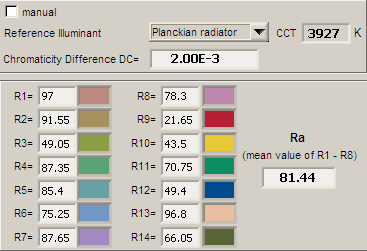CRI of the light of this lightbulb.

The value of 81 is higher than 80 which is considered a minimum value for indoor usage.

Note: the chromaticity difference is 0.002 indicates the distance to the Planckian Locus. Its value is lower than 0.0054, which means that the calculated CRI result is meaningful.

### Voltage dependency

The dependency of a number of lamp parameters on the lamp voltage is determined. For this, the lamp voltage has been varied and its effect on the following lamp parameters measured: illuminance E_v [lx], color temperature CT or correlated color temperature CCT [K], the lamppower P [W] and the luminous efficacy [lm/W].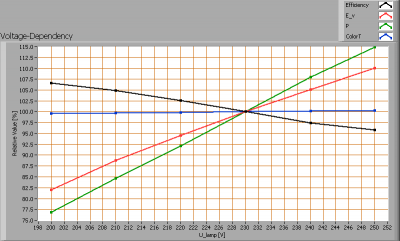Lamp voltage dependencies of certain light bulb parameters, where the value at 230 V is taken as 100 %.

The consumed power and the illuminance vary largely when the voltage varies between 200 – 250 V. This variation is linear.

When the voltage at 230 V varies with + and – 5 V, then the illuminance varies with less than + and – 2.5 %, which will not be visible when the voltage changes abruptly.

### Warm up effects

After switch on of a cold lamp, the effect of heating up of the lamp is measured on illuminance E_v [lx], color temperature CT or correlated color temperature CCT [K], the lamppower P [W] and the luminous efficacy [lm/W].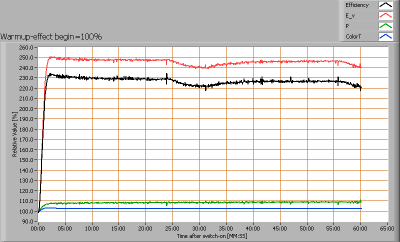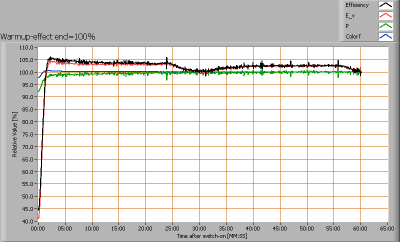Effect of warming up on different light bulb parameters. At top the 100 % level is put at begin, and at bottom at the end.

After three minutes the light bulb gives the maximum light output. Visitble are the small variations in illuminance, due to the ambient temperature variation. FL tubes are sensitive to ambient temperature variations, here a change of 2.5 deg in ambient temperature results in 2.5 % variation of the light output.